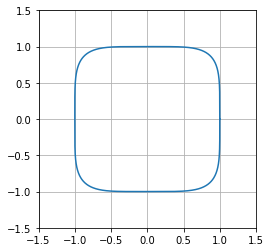# Vector Norms¶

## Computing norms by hand¶

$p$-norms can be computed in two different ways in numpy:

In :
import numpy as np
import numpy.linalg as la

In :
x = np.array([1.,2,3])


First, let's compute the 2-norm by hand:

In :
np.sum(x**2)**(1/2)

Out:
3.7416573867739413

Next, let's use numpy machinery to compute it:

In :
la.norm(x, 2)

Out:
3.7416573867739413

Both of the values above represent the 2-norm: $\|x\|_2$.

## About the $\infty$-norm¶

Different values of $p$ work similarly:

In :
np.sum(np.abs(x)**5)**(1/5)

Out:
3.077384885394063
In :
la.norm(x, 5)

Out:
3.077384885394063

The $\infty$ norm represents a special case, because it's actually (in some sense) the limit of $p$-norms as $p\to\infty$.

Recall that: $\|x\|_\infty = \max(|x_1|, |x_2|, |x_3|)$.

Where does that come from? Let's try with $p=100$:

In :
x**100

Out:
array([1.00000000e+00, 1.26765060e+30, 5.15377521e+47])
In :
np.sum(x**100)

Out:
5.153775207320113e+47

Compare to last value in vector: the addition has essentially taken the maximum:

In :
np.sum(x**100)**(1/100)

Out:
3.0

Numpy can compute that, too:

In :
la.norm(x, np.inf)

Out:
3.0

## Unit Balls¶

Once you know the set of vectors for which $\|x\|=1$, you know everything about the norm, because of semilinearity. The graphical version of this is called the 'unit ball'.

We'll make a bunch of vectors in 2D (for visualization) and then scale them so that $\|x\|=1$.

In :
alpha = np.linspace(0, 2*np.pi, 2000, endpoint=True)
x = np.cos(alpha)
y = np.sin(alpha)

vecs = np.array([x,y])

p = 5
norms = np.sum(np.abs(vecs)**p, axis=0)**(1/p)
norm_vecs = vecs/norms

import matplotlib.pyplot as pt
%matplotlib inline
pt.grid()
pt.gca().set_aspect("equal")
pt.plot(norm_vecs, norm_vecs)
pt.xlim([-1.5, 1.5])
pt.ylim([-1.5, 1.5])

Out:
(-1.5, 1.5)